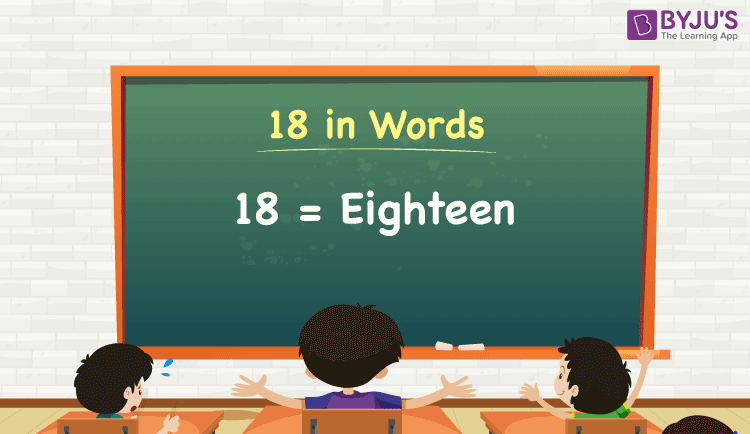# 18 in Words

We can write 18 in words as Eighteen. With the help of a place value chart, we can easily derive the number 18 in words. If you have 18 candies, then you can say, “I have Eighteen candies with me”. However, 18 is a cardinal number since it denotes some specific amount.

 18 in words: Eighteen Eighteen in numerical form: 18

18 in English Words

We generally write numbers in words using the English alphabet. Thus, we can read 18 using the words in English as “Eighteen”.## How to Write 18 in Words?

18 is a two-digit number so let’s make a place value chart with two columns as shown below.

 Tens Ones 1 8

Here, ones = 8 and tens = 1.

So, let us expand these digits as per the place values and combine the results to get the final number.

1 × Tens + 8 × Ones

= 1 × 10 + 8 × 1

= 10 + 8

= 18

= Eighteen

Therefore, 18 in words = Eighteen.

Interesting way of writing 18 in words:

1 = One

8 = Eight

10 = Ten

10 + 8 = 18

Ten + Eight = Eighteen

Thus, the word form of 18 is Eighteen.

### Facts About the Number 18

18 is a natural number that is the successor of 17 and the predecessor of 19.

18 in words – Eighteen

Is 18 an odd number? – No

Is 18 an even number? – Yes

Is 18 a prime number? – No

Is 18 a composite number? – Yes

Is 18 a perfect square number? – No

Is 18 a perfect cube number? – No

How do you write 18 in Roman numerals? – XVIII

### 1 to 20 in Words

 1 in words = One 11 in words = Eleven 2 in words = Two 12 in words = Twelve 3 in words = Three 13 in words = Thirteen 4 in words = Four 14 in words = Fourteen 5 in words = Five 15 in words = Fifteen 6 in words = Six 16 in words = Sixteen 7 in words = Seven 17 in words = Seventeen 8 in words = Eight 18 in words = Eighteen 9 in words = Nine 19 in words = Nineteen 10 in words = Ten 20 in words = Twenty

Get the conversion of some particular numbers to words here.

21 in words = Twenty-one

27 in words = Twenty-seven

30 in words = Thirty

35 in words = Thirty-five

40 in words = Forty

50 in words = Fifty

90 in words = Ninety

## Frequently Asked Questions on 18 in Words

Q1

### How do you write 18 in words?

The spelling of 18 in words is Eighteen, which means 18 in words can be expressed as Eighteen.

Q2

### How do you write Rs. 18 in words?

We can write Rs. 18 in words as Eighteen rupees.

Q3

### Write the value of 11 + 7 in words.

11 + 7 = 18
Eleven + Seven = Eighteen
Therefore, 11 + 7 is equal to Eighteen.